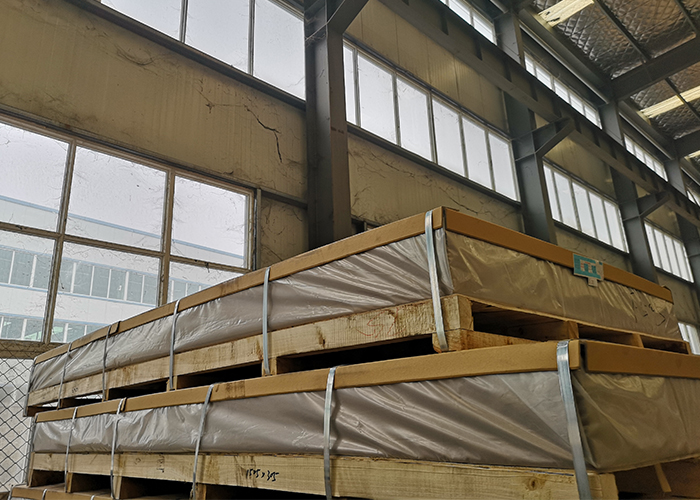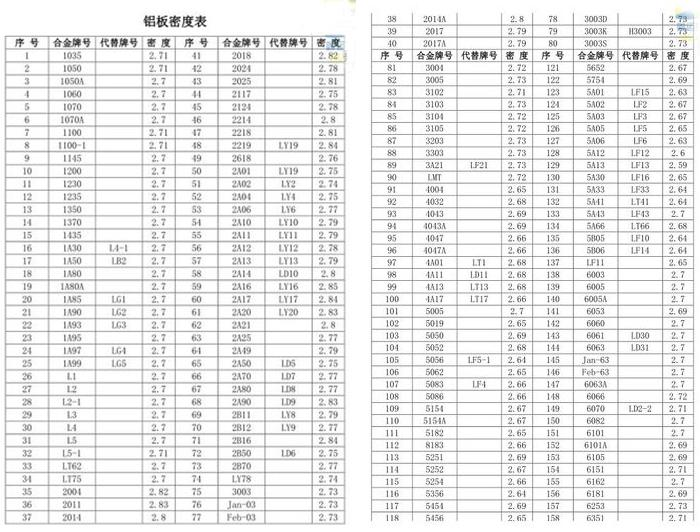Welcome to the web of Haomei Aluminum

# Anodized aluminium sheet 6mm wight

### December 13, 2019aluminium sheetanodized aluminium sheetanodized aluminium sheet 6mm wight

The phrase “anodized aluminium sheet 6mm wight” refers to the weight of a 6mm anodized aluminium sheet. Generally speaking, the weight of a piece aluminium sheet is up to not only its thickness but also its sizes and density.

The formula for calculating the wight of an anodized alu sheet is: thickness*size*density. The density of aluminum alloy sheets are more or less the same with pure aluminium: 2.7g/cm³. Thus you can simply obtain the anodized aluminium sheet 6mm wight by using the formula above. Here you need to measure the length and width of the sheet first. If you are not sure about a sheet’s thickness, you need to measure the thickness, too.Here we give a full introduction to steps of getting the weight of an anodized aluminium sheet or aluminium sheets of other types.

Step One—Measure

If you have a vernier caliper, use it to measure the thickness of the aluminum plate. This is the most accurate method;

It doesn’t matter if you don’t have it, especially with aluminum plates of 4mm or more. Place the aluminum plate on a flat surface, measure the thickness of the aluminum plate with a ruler, and record the measurement result.

The length and width can be measured with a tape measure. Measure along both sides of the aluminum plate and record the results.

Step Two—Calculate the Wight

1.Calculation formula

Aluminium sheet plate weight = aluminum plate thickness * width * length * density

2.Aluminum sheet density

The density of pure aluminum is 2.7 × 10kg / m³ or 2.7g / cm³;

Densities of different aluminium alloys are as shown in the tables. The few Chinese characters will not stop you from reading the tales.3. Examples

Assume the volume V of an aluminum plate with a length of 728mm, a width of 380mm, and a thickness of 3.0mm: V = 3.0mm * 0.728 * 0.38

The weight of the aluminum plate: V * 2.7g / cm³ = 2.24kg1.

Things Worth Attention

The weight of an aluminium sheet metal obtained by theoretical calculations performed strictly according to the table is often lighter than its actual weight, because the actual thickness and size of the aluminum plate are not so accurate, and the aluminum plate will also affect the actual weighing after being coated. Thus the theoretical calculations provide only a rough guide.

A more accurate way would be measure the weight of a pile of finished anodized aluminum sheets 6mm and then divide the result by the piece number of the sheets, and you will get an anodized aluminium sheet 6mm wight of the pile.